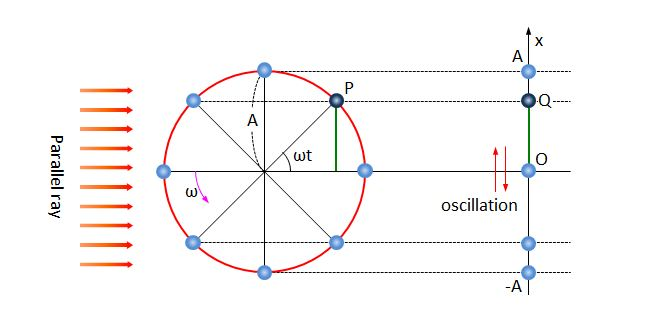# Harmonic oscillatorWhen we light a parallel ray on the object($P$) which is in uniform circular motion with angular velocity $\omega$, the motion of its shadow($Q$) on the right side screen is a harmonic oscillation. What are the displacement(vector) and period, respectively, of the harmonic oscillation of the shadow($Q$) at time $t$ as shown above?

×